# GSEB Solutions Class 8 Maths Chapter 5 Data Handling InText Questions

Gujarat Board GSEB Textbook Solutions Class 8 Maths Chapter 5 Data Handling InText Questions and Answers.

## Gujarat Board Textbook Solutions Class 8 Maths Chapter 5 Data Handling InText Questions

Try These (Page 71)

Question 1.
If we change the position of any of the bars of a graph, would it change the information being conveyed? Why?
Solution:
If the height of a bar remains unchanged, then changing of its position does not change the information being conveyed.

Try These (Page 71)

Question 1.
Draw an appropriate graph to represent the given information?

1.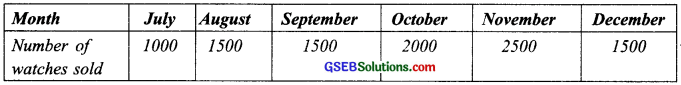2.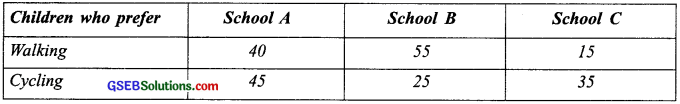3.
Percentage wins in ODI by 8 top cricket teams?Solution:
Note: A bar graph showing two sets of data simultaneously is called a double – bar graph. It is useful for the comparison of the data.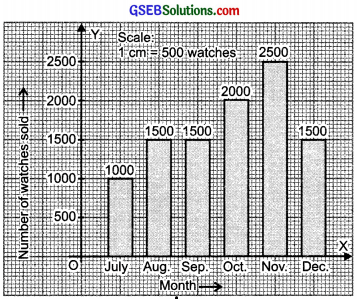1. To represent the given data by a bar-graph, draw two axes perpendicular to each other. Now, represent ‘Months’ on OX and ‘Number of watches sold’ on OY. Erect rectangles of the same width. The heights of the rectangles are proportional to number of watches, using a suitable scale:
Here, Since scale is 1 cm = 500 watches
Since 500 watches = 1 cm
∴ 1000 watches = 2 cm
1500 watches = 3 cm
2000 watches = 4 cm
2500 watches = 5 cm

2.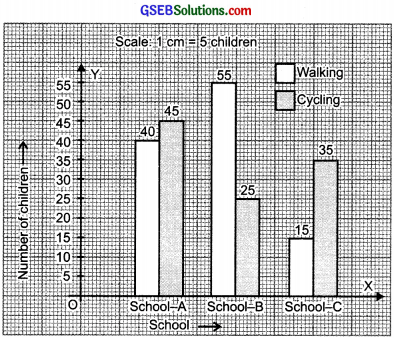Since, a comparison of two activities (walking and cycling) is to be represented, therefore a double-graph is drawn by taking the schools along x-axis and number of children along y-axis, using a scale of 1 cm = 5 children.

3.To compare the percentage win in ODI achieved by various teams, we represent the data by a double-bar graph. We represent the teams along the x-axis and their ‘percentage win’ along y-axis, using the scale 1 cm = 5%.

Try These (Page 72)

Question 1.
A group of students were asked to say which animal they would like most to have as a pet. The results are given below:
dog, cat, cat, fish, cat, rabbit, dog, cat, rabbit, dog, cat, dog, dog, dog, cat, cow, fish, rabbit, dog, cat, dog, cat, cat, dog, rabbit, cat, fish, dog.
Make a frequency distribution table for the same.
Solution:
Using tally-marks, we have:Try These (Page 73)

Question 1.
Study the following frequency distribution table and answer the questions given below?
Frequency distribution of daily income of 550 workers of a factory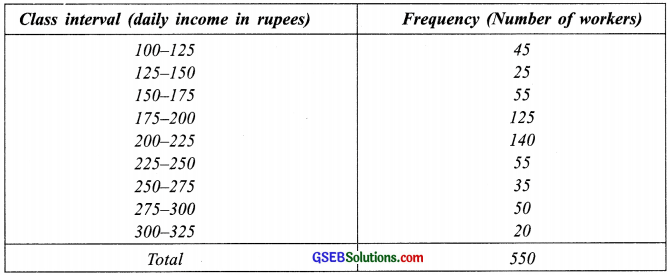1. What is the size of the class intervals?
2. Which class has the highest frequency?
3. Which class has the lowest frequency?
4. What is the upper limit of the class interval 250-275?
5. Which two classes have the same frequency?

Solution:

1. Class size = [Upper class limit] – [Lower class limit] = 125 – 100 = 25.
2. The class 200-225 is having the highest frequency (which is 140).
3. The class 300-325 is having the lowest frequency (which is 20).
4. The upper limit of the class interval 250-275 is 275.
5. The classes (150-175) and (225-250) are having the same frequency (which is 55).Question 2.
Construct a frequency distribution table for the data on weights (in kg) of 20 students of a class using intervals 30 – 35, 35 – 40, and so on?
40, 38, 33, 48, 60, 53, 31,
46, 34, 36, 49, 41, 55, 49,
65, 42, 44, 47, 38, 39
Solution:
Lowest observation = 31
Highest observation = 65
Class intervals: 30 – 35, 35 – 40, 40 – 45, ……
The frequency distribution table for the above data can be: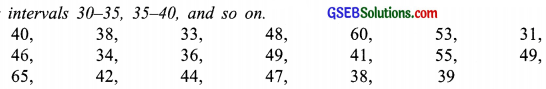Try These (Page 75)

Question 1.
Observe the histogram, figure and answer the questions given below:1. What information is being given by the histogram?
2. Which group contains maximum girls?
3. How many girls have a height of 145 cm and more?
4. If we divide the girls into the following three categories, how many would there be in each?

150 cm and more – Group A
140 cm to less than 150 cm – Group B
Less than 140 cm – Group C

Solution:

1. The above histogram represents the height (in cms) of girls of Class VIII.
2. The group 140-145 contains maximum number of girls (which has as much as 7 girls).
3. 7 girls (= 4 + 2 + 1) have a height of 145 cm and more.
4. Number of girls in:-

Group A: 150 cm and more = 2 + 1 = 3 girls
Group B: 140 cm to less than 150 cm = 7 + 4 = 11 girls
Group C: Less than 140 cm = 1 + 2 + 3 = 6 girls

Note:
The broken lineis used along the horizontal line to indicate that we are not showing the numbers between 0 and 125.

Try These (Page 78)

Question 1.
Each of the following pie charts gives you a different piece of information about your class. Find the fraction of the circle representing each of these information?Solution:
1. Fraction of the circle representing the ‘girls’ 50% = $$\frac{50}{100}$$ = $$\frac{1}{2}$$
Fraction of the circle representing the ‘boys’ 50% = $$\frac{50}{100}$$ = $$\frac{1}{2}$$

2. Fraction of the circle representing ‘walk’ 40% = $$\frac{40}{100}$$ = $$\frac{2}{5}$$
Fraction of the circle representing ‘bus or car’ 40% = $$\frac{40}{100}$$ = $$\frac{2}{5}$$
Fraction of the circle representing ‘cycle’ 20% = $$\frac{20}{100}$$ = $$\frac{1}{5}$$

3. Fraction of the circle representing those who love mathematics
= (100 – 15)% = $$\frac{100-15}{100}$$ = $$\frac{85}{100}$$ = $$\frac{17}{20}$$
Fraction of the circle representing those who hate mathematics = 15% = $$\frac{15}{100}$$ = $$\frac{3}{20}$$Question 2.
Answer the following questions based on the pie chart given?

1. Which type of programmes are viewed the most?
2. Which two types of programmes have number of viewers equal to those watching sports channels?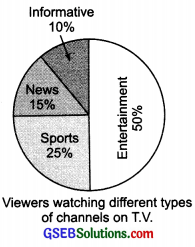Solution:
From the given pie chart, we haveObviously,

1. The entertainment programmes are viewed the most.
2. The news and informative programmes have the equal number of viewers.

Try These (Page 81)

Question 1.
Draw a pie chart of the data given below?
The time spent by a child during a day.
Sleep – 8 hours
School – 6 hours
Home work – 4 hours
Play – 4 hours
Others – 2 hours
Solution:
First we find the central angle corresponding to the given activities.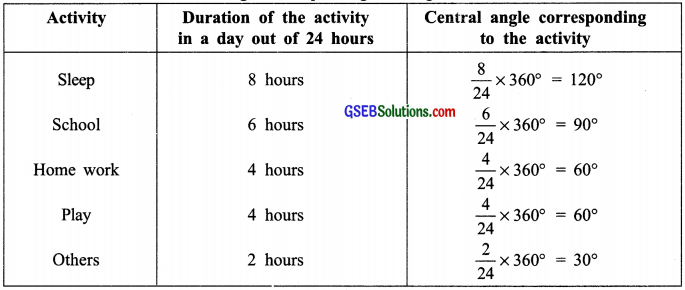Now, the required pie chart is given below:Try These (Page 81)

Question 1.
Which form of graph would be appropriate to display the following data?
Production of food gains of a stateSolulution:
A bar graph will be an appropriate representation of the above data.Question 2.
Choice of food for a group of peopleSolution:
A circle graph (or a pie chart).

Question 3.
The daily income of a group of a factory workers.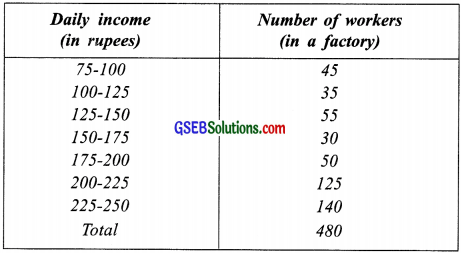Solution:
A histogram would be appropriate representation of the above data.

Try These (Page 83)

Question 1.
If you try to start a scooter, what are the possible outcomes?
Solution:
It may start. It may not start.

Question 2.
When a die is thrown, what are the possible outcomes?
Solution:
The possible outcomes are: 1, 2, 3, 4, 5 or 6.Question 3.
When you spin the wheel shown, what are the possible outcomes? List them? (Outcome here means the sector at which the pointer stops).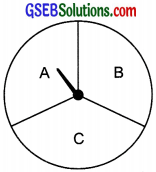Solution:
The possible outcomes are A, B or C.

Question 4.
You have a bag with five identical balls of would get?
Solution:The possible outcomes are: W, R, B, G or Y.

Try These (Page 84)

Question 1.
In throwing a die:
(a) Does the first player have a greater chance of getting a six?
(b) Would the player who played after him have a lesser chance of getting a six?
(c) Suppose the second player got a six. Does it mean that the third player would not have a chance of getting a six?
Solution:
(a) No
(b) No
(c) No

Try These (Page 86)

Question 1.
Suppose you spin the wheel.

1.
(i) List the number of outcomes of getting a green sector and not getting a green sector on this wheel.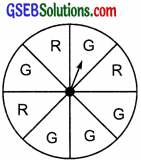(ii) Find the probability’ of getting a green sector.
(iii) Find the probability of not getting a green sector.
Solution:
(i) Number of outcomes of getting a green sector = 5
Number of outcomes of not getting a green sector = 3

(ii) ∵ The total number of equally likely outcomes = 8
Number of outcomes of getting a green sector = 5
Probability of getting a green sector = $$\frac{5}{8}$$

(iii) Probability of not getting a green sector = $$\frac{3}{8}$$